# Maximum principle

(diff) ← Older revision | Latest revision (diff) | Newer revision → (diff)

discrete

The Pontryagin maximum principle for discrete-time control processes. For such a process the maximum principle need not be satisfied, even if the Pontryagin maximum principle is valid for its continuous analogue, obtained by replacing the finite difference operator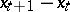by the differential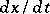. For example, consider the optimal control problem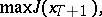(1)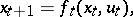(2)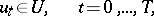(3)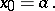(4)

This can be treated as a standard problem on an extremum in the presence of constraints. Then the condition for optimality of a trajectory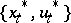can be obtained with the help of the Lagrange function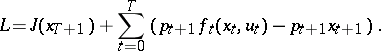Here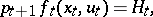by analogy with the continuous case, is called the Hamilton function. Let,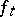,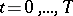, be differentiable functions with respect to all variables and letbe a bounded closed set. Then for a solutionof (1)–(4) to be optimal it is necessary that there exist Lagrange multipliers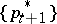such that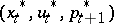is a stationary point of the Lagrange function, that is, at this point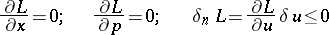for all admissible variations of the control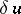. The first condition leads to the dynamical equations of the discrete process (2) and the initial condition (4). The second leads to the boundary condition and the conjugate system for the impulses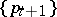: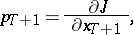The third condition leads to a condition on the first variation of the Hamilton function: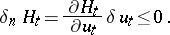(5)

However, (5) does not imply that the Hamilton function attains its maximum at the optimal control,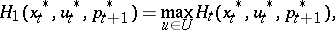over all controls satisfying the constraint (3); it shows that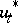is a stationary point of the Hamilton function. If the first variation of the Hamilton function,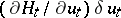, is zero (this holds, in particular, whenis an interior point or when there are admissible variationsof the control atorthogonal to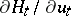), then the nature of the stationary point is determined by the successive terms in the expansion:Examples have been constructed in whichis a local maximum, a local minimum and even a saddle point of the Hamilton function. Therefore, in general, the maximum principle does not hold for discrete systems.

For systems which are linear in the phase variables,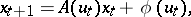or in the controls,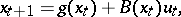under the additional condition of linearity of the objective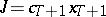in the first case, or convexity ofin the second case, the maximum principle is satisfied (see ).

If the optimal control problem for a linear discrete system is treated as a linear programming problem (see , ), then its dual discrete-time dynamical problem may be obtained. The conjugate system for the impulses gives the dynamical equation for the dual problem. On an optimal trajectory not only the objective but also the Hamilton functions of dual dynamical systems coincide.

How to Cite This Entry:
Maximum principle. Encyclopedia of Mathematics. URL: http://encyclopediaofmath.org/index.php?title=Maximum_principle&oldid=14552
This article was adapted from an original article by Yu.P. Ivanilov (originator), which appeared in Encyclopedia of Mathematics - ISBN 1402006098. See original article